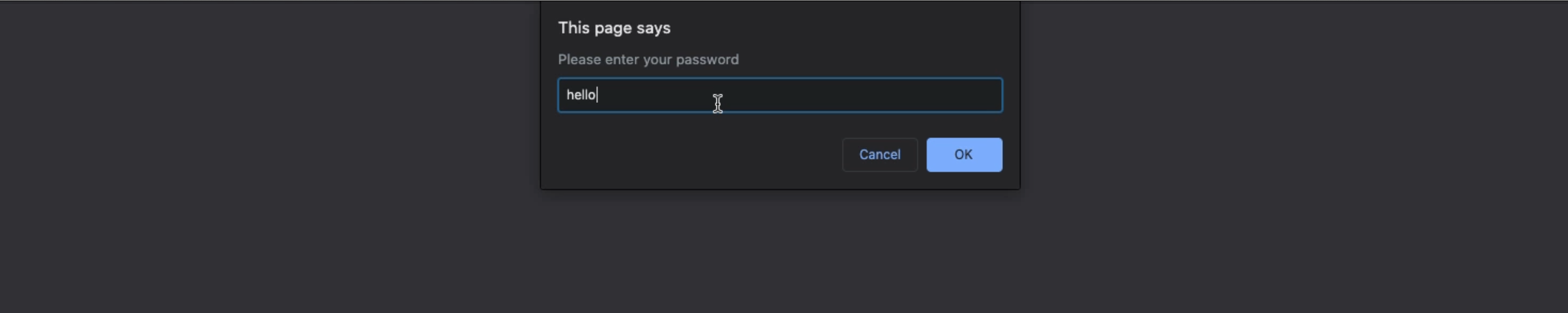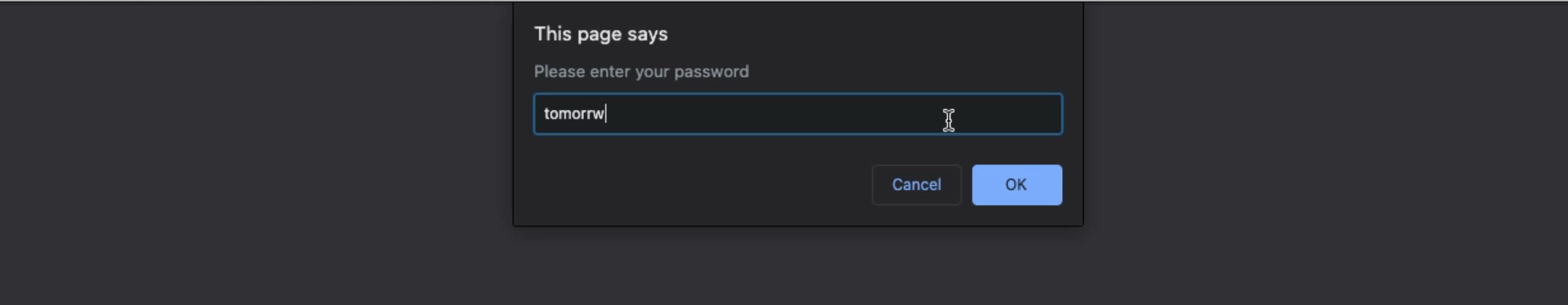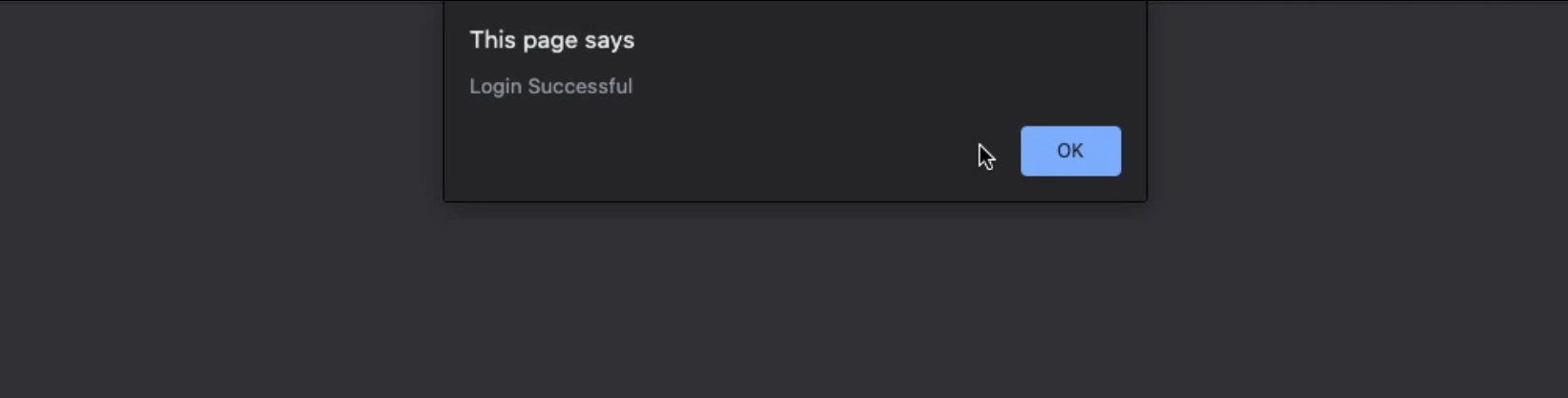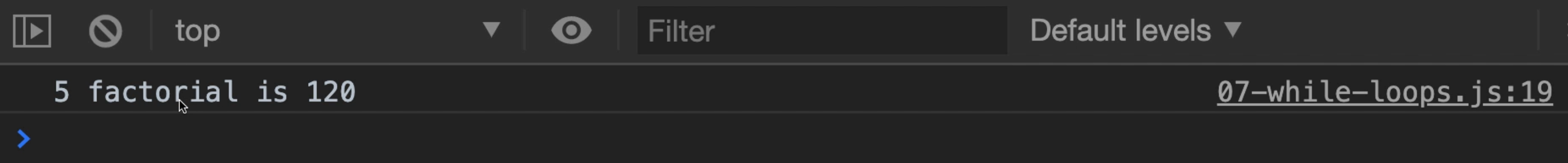Transcripted Summary

In the previous video, we focused on using `for` loops, and in this video, we're going to see how we can use a different type of loop.

You see, `for` loops are really helpful when you have a predefined number of steps that you want to take, like when you're using a `for` loop to go through each item of an array, for example.

But what if you don't know how many times you want a loop to run, but instead, you have a better idea about when you want it to stop?

Let's take user input. We might want to ask the user to repeat entering information until they give you the correct information.

Let's take the specific example of asking a user to enter a password.

You would probably repeat that step until they entered the right password.

Let's actually write some code to accomplish this.

### # While loops

A `while` loop starts with the `while` keyword.

Like with other programming syntax, we have to let JavaScript know what to expect.

After you type the `while` keyword, then you put parentheses `()`.

Inside the parentheses, you put the condition in which to keep running the loop.

So this means, as long as the condition is true, the loop will continue.

Let's define some variables in file `07-while-loops.js`.

For our condition, we'll say, `while` the `userGuess` does not equal `password`.

So as long as the `userGuess` does not equal `password`, we want to execute the body of this loop. Let's write some code for that.

We use curly braces `{}` to group our code together.

We'll use the `prompt()` to support.

``````let password = "tomorrow";
let userGuess = "";

}

``````

We have our `for` loop and then we have our `userGuess` variable, and we say the user guess will equal whatever value from the prompt.

When we're done, we want to let the user know that they've successfully logged in.

In this case, we can use an `alert`.

Okay friends, let's see what this code looks like in the browser.

We have our webpage, and I'm going to enter any type of value.If I enter "hello", it's going to keep asking me. The password is actually "tomorrow", but I'm not going to spell tomorrow correctly.Okay, now I'll spell it correctly.Then we have a "Login Successful".

Let's look at this loop one more time.

When we first get to `line 4`, `userGuess` is empty string `""` and `password` is still `tomorrow`.

Empty string does not equal `password`, so we actually go inside of the loop.

Then, using the `prompt` in JavaScript, we send up a popup box that the user can enter information.

Whatever they enter gets stored back into `userGuess`.

And then the loop loops around again, meaning it repeats itself.

And then it checks to see, well, what's the value of `userGuess`, and if it doesn't equal `password`, we want to do this loop once more.

And you can continue to do this, until you have your condition to stop the loop.

In our case, our condition specifically is when `userGuess` equals `password` - the loop will stop.

I'm going to give you a warning here.

But I wanted to show you how this works.

### # Do while loops

There's another variation of the `while` loop that we can look into.

That is called `do while`.

The syntax looks a little bit different, but it works very similar to a `while` loop.

While vs Do While

The important difference is that the `do while` loop will run at least once, whereas with the `while` loop, there's no guarantee that it will run at least once.

One example that could be helpful to thinking about a `while` loop is to calculate the factorial of a number. The factorial of five. Let's write it out.

``````// 5 * 4 * 3 * 2 * 1
``````

Now, let's write this with a `do while`.

The first step is to write the keyword `do`, then you put your curly braces `{}`.

Now, next to the closing curly brace, this is where you put your `while` statement.

So say, `do` this `while` something happens.

Well, in our case, we're doing a factorial. So let's make some variables.

``````let password = "tomorrow";
let userGuess = "";

}

// 5 * 4 * 3 * 2 * 1

let factorial = 1;
let number = 5;

do {

} while (number > 0);
``````

Now, our condition here is, `while` number is greater than `0`, we want to do this work.

And be mindful, on `line 15`, there's a semicolon there. You want to have that.

Let's put the logic.

We'll say `factorial` equals the current `factorial` times the `number`.

Then we will subtract one using `--`.

So this will start at `5` and go all the way down until this condition is no longer valid.

And this condition is no longer valid when the number is no longer greater than `0`.

Let's `console.log` a message.

``````let password = "tomorrow";
let userGuess = "";

}

// 5 * 4 * 3 * 2 * 1

let factorial = 1;
let number = 5;

do {
factorial = factorial * number;
number--
} while (number > 0);

console.log(number + " factorial is " + factorial);
``````

Okay. Let's try this out in the browser.

We still have a `while` loop here, so I'm going to actually stop the `while` loop from happening just so we can see this work.

I'll comment it out, but still save our code.

And then, I'll just say `original` equals `number`.

Now, because we're updating that number, so then we can change this code to be `original`.

``````let password = "tomorrow";
let userGuess = "";

// while (userGuess !== password) {
// }

// 5 * 4 * 3 * 2 * 1

let factorial = 1;
let number = 5;
let original = number;

do {
factorial = factorial * number;
number--
} while (number > 0);

console.log(original + " factorial is " + factorial);
``````

Let's save it and go to the browser console.And 5 factorial is 120.

So we can see, no matter what, this code will run at least once.

That's when a `do while` loop is very effective.

It's when you need your code to run at least once, and then it checks the condition after, whereas, a `while` loop will check the condition first.

Loops are incredibly helpful structures that allow you to repeat blocks of code.

We've seen how we can use `for` loops if we know exactly how many times you want to run a loop.

Or we can use a `while` loop if we have a condition that we need to meet before we can stop the loop.